# High Cycle Fatigue

High cycle fatigue (HCF) occurs at relatively large numbers of cycles. The arbitrary classification between high cycle fatigue and low cycle fatigue (LCF) is considered to be roughly 10,000 cycles, where stress is relatively low and deformation is primarily elastic.  In general high cycle fatigue is caused by alternating stresses in the elastic or slightly inelastic range, while low cycle fatigue is normally in the inelastic or plastic range which exhibits large strains; the exact number of cycles depends on the properties of the metal.A study in 1992 conducted by the Scientiﬁc Advisory Board of the US Air Force targeted high cycle fatigue as the single biggest cause of turbine engine failures in military aircraft.  High cycle fatigue results in rapid, essentially unpredictable failures due to fatigue-crack propagation under ultrahigh frequency loading.  Furthermore, cracking often initiates from small defects which are associated with damage caused by a variety of drivers, including fretting and impacts by foreign objects.

### Stress-cycle (S-N) curve

In high cycle fatigue situations, materials performance is commonly characterized by an S-N curve, or stress-cycle curve, or fatigue curve, also known as a Wöhler curve. This is a graph of the magnitude of a cyclic stress (S) against the logarithmic scale of cycles to failure (N).

The S-N curve in the high-cycle region is sometimes described by the Basquin equation: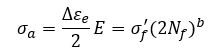or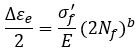where

• σa is the elastic stress amplitude
• Δεe /2 is the elastic strain amplitude
• 2Nf is the number of reversals to failure (Nf cycles)
• E is modulus of elasticity
• σ’f is fatigue strength coefficient.  A fairly good approximation is σ’f = σf where σf is fracture stress with necking corrections.  For steels with hardness below 500 HB, σf = UTS + 50 ksi. Other values in estimating σ’f include:

σ’f = 1.09 σf
σ’f = 0.92 σf (b = -0.12)
σ’f = 1.15 σf (b = -0.12)

• b is the fatigue strength exponent, commonly in the range -0.12 < b < -0.05 with an average of -0.085

The Fatigue+ software provides a material database including extensive fatigue data for carbon steels, low alloy steels, high alloy steels, aluminum alloys, as well as cast metals and welds.  The user can edit the database or add materials to the database.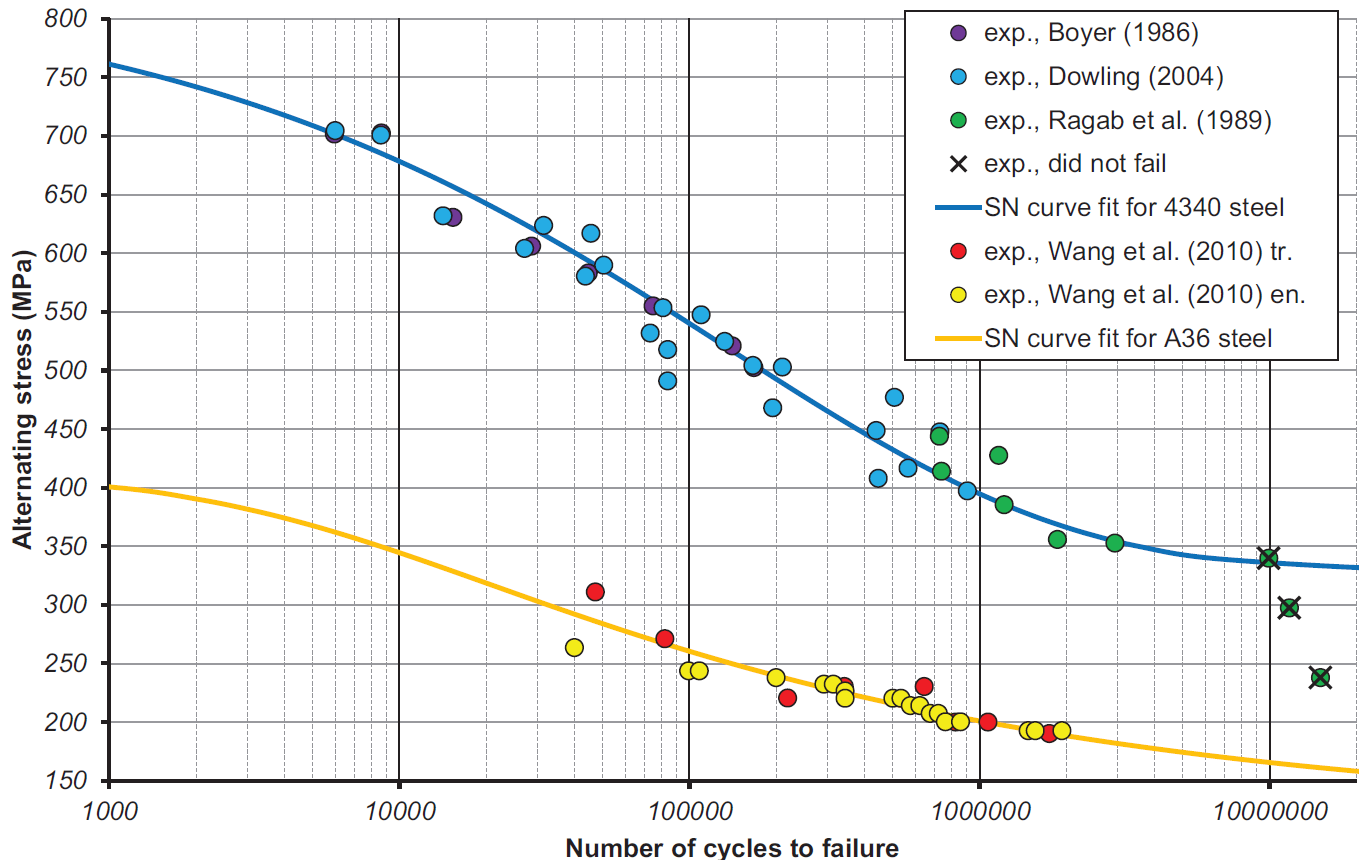S-N curves of ASTM A-36 and AISI 4340 based on HCF data

S-N curves are derived from tests on samples of the material to be characterized (often called coupons) where a regular sinusoidal stress is applied by a testing machine which also counts the number of cycles to failure. This process is sometimes known as coupon testing. Each coupon test generates a point on the plot though in some cases there is a runout where the time to failure exceeds that available for the test. Analysis of fatigue data requires techniques from statistics, especially survival analysis and linear regression.

The progression of the S-N curve can be influenced by many factors such as corrosion, temperature, residual stresses, and the presence of notches. The Goodman-Line is a method used to estimate the influence of the mean stress on the fatigue strength.

### Probabilistic nature of fatigue

As coupons sampled from a homogeneous frame will display a variation in their number of cycles to failure, the S-N curve should more properly be a Stress-Cycle-Probability (S-N-P) curve to capture the probability of failure after a given number of cycles of a certain stress. Probability distributions that are common in data analysis and in design against fatigue include the log-normal distribution, extreme value distribution, Birnbaum–Saunders distribution, and Weibull distribution.In practice, a mechanical part is exposed to a complex, often random, sequence of loads, large and small. In order to assess the safe life of such a part:

2. A histogram of cyclic stress is created from the rainflow analysis to form a fatigue damage spectrum;
3. For each stress level, the degree of cumulative damage is calculated from the S-N curve; and
4. The effects of the individual contributions are combined using an algorithm such as Miner’s rule.

Since S-N curves are typically generated for uniaxial loading, some equivalence rule is needed whenever the loading is multiaxial. For simple, proportional loading histories (lateral load in a constant ratio with the axial), Miner’s rule may be applied. For more complex situations, such as nonproportional loading, critical plane analysis should be used.

#### Miner’s Rule

In 1945, M A Miner popularized a rule that had first been proposed by A. Palmgren in 1924. The rule, variously called Miner’s rule or the Palmgren-Miner linear damage hypothesis, states that where there are k different stress magnitudes in a spectrum, Si (1 ≤ ik), each contributing ni(Si) cycles, then if Ni(Si) is the number of cycles to failure of a constant stress reversal Si (determined by uni-axial fatigue tests), failure occurs when:C is experimentally found to be between 0.7 and 2.2. Usually for design purposes, C is assumed to be 1.0. This can be thought of as assessing what proportion of life is consumed by a linear combination of stress reversals at varying magnitudes.

Because of the great convenience of Miner’s rule, it is widely used and well accepted in the industry.

### Paris’ Law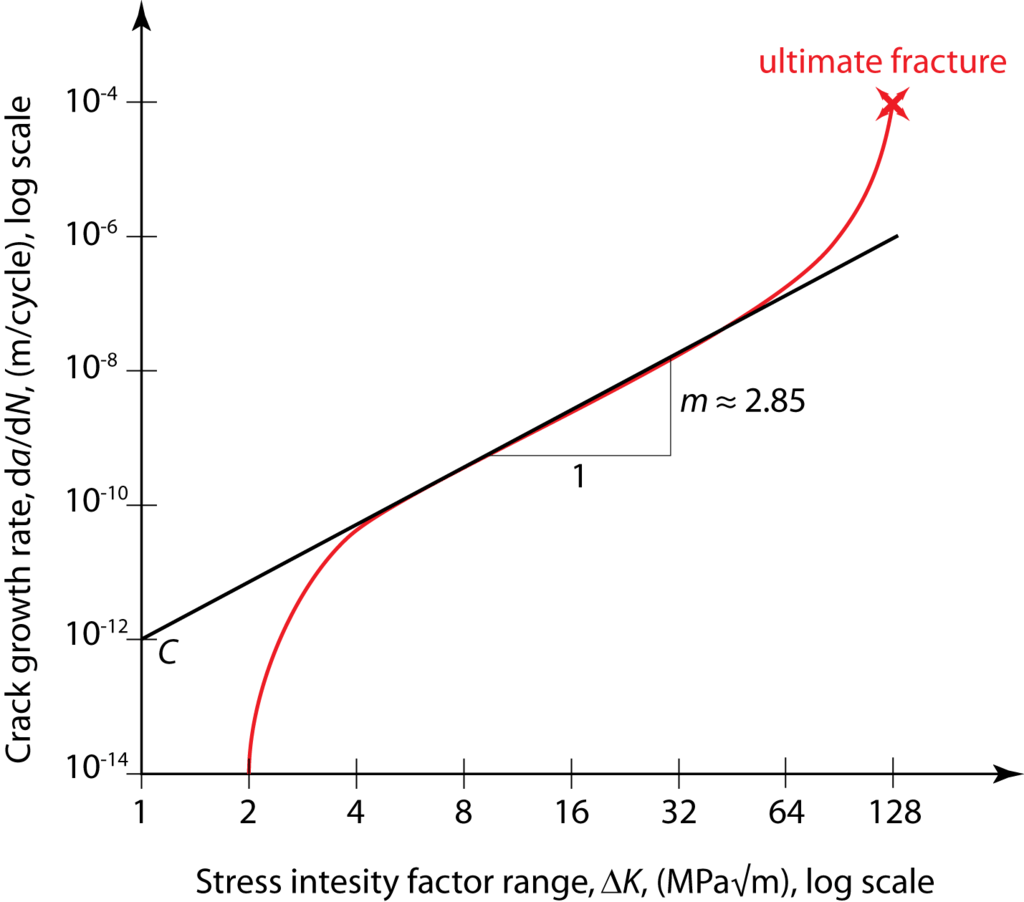Typical fatigue crack growth rate graph

In fracture mechanics, Anderson, Gomez, and Paris derived relationships for the stage II crack growth with cycles N, in terms of the cyclical component ΔK of the Stress Intensity Factor Kwhere a is the crack length and m is typically in the range 3 to 5 (for metals), which states that the rate of crack growth with respect to the cycles of load applied is a function of the stress intensity factor; this is named Paris’ law.

This relationship was later modified (by Forman, 1967) to make better allowance for the mean stress, by introducing a factor depending on (1-R) where R= min stress/max stress, in the denominator.

### Mean Stress Correction

High cycle fatigue is sensitive to mean stresses.  In the software Fatigue+, the following options are available for mean stress correction:

For Basquin model, select Soderberg, Goodman, Gerber,Morrow, Smith-Watson-Topper, or None.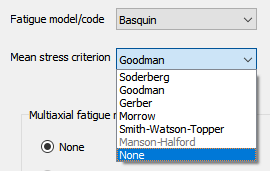For Basquin-Coffin-Manson relation, select Morrow, Smith-Watson-Topper, Manson-Halford, or None. (See Low Cycle Fatigue)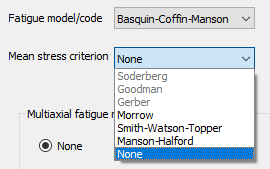In the design code based fatigue models, the mean stress correction is often incorporated into the models in compliance with corresponding design codes.